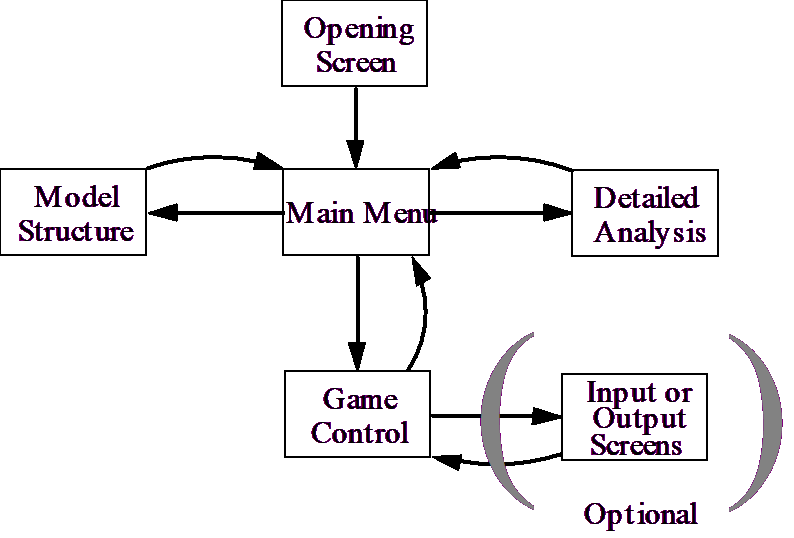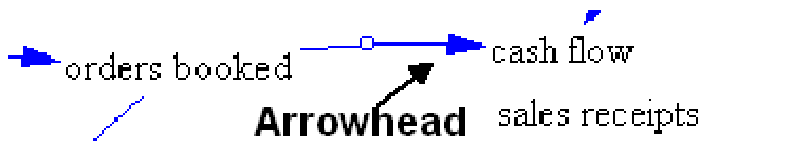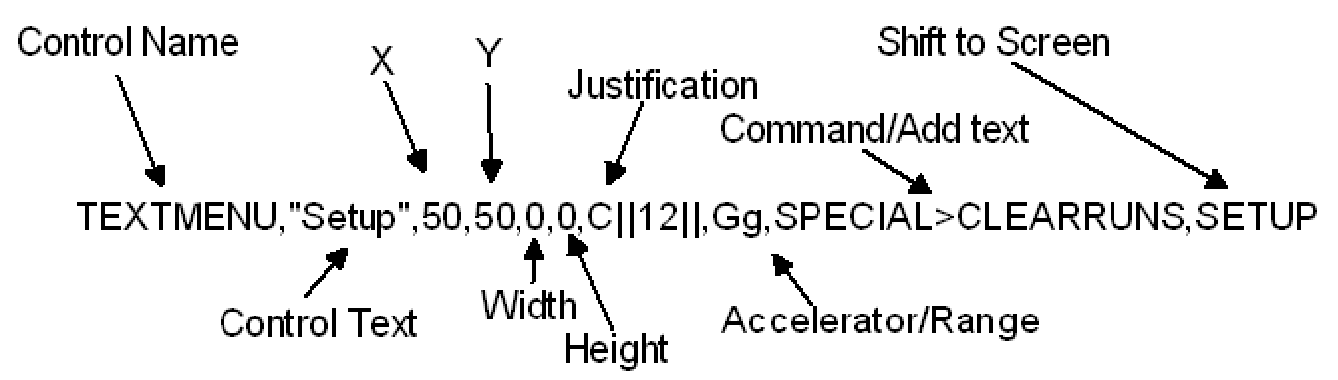# Vensim Help

 Navigation: Reference Guide > The Vensim Modeling Language Data Equations

Data have two purposes in models.  First, they can be used to compare the behavior of models to that of real systems.  Second, they can drive model variables that do not warrant explicit endogenous modeling.  No distinction is made between the two types of data, but actual practice often places the two types into separate models or files.  We will touch on this briefly below.

The equations defining data are largely the same as those defining other variables, except that a colon equal := is used instead of an equal sign.  Data equations also have some special features that determine how the data will be used if they are referenced elsewhere in the model as exogenous inputs.  There are two ways to mark variables as Data variables.

 1 Do not write any equation.  If something is used it will be marked as not defined and thereafter treated as exogenous.  This approach is useful only in the Text Editor.
 2 In the Equation Editor mark a variable as of type Data, possibly with an equation.  In the Text Editor enter the variable name with no equation,
 fixed cost data  ~~|

or write an equation using :-.

 cost data := variable cost data + fixed cost data ~~|

Only other data and constants may appear on the right-hand side of the last type of equation.  These must either be computed using other  :=  equations, or be read in directly as raw data.

When data variables are used as exogenous inputs, they will have values at distinct time points only.  Between these time points it is necessary to fix values for the exogenous inputs.  There are four ways of doing this.

 • Interpolating a value (the default).
 • Holding a value when it is passed until a new value is found.
 • Looking forward to the next value and using it.
 • Marking data as missing when not available.

These are invoked using keywords, as in the following example:

production ahead :LOOK FORWARD: := planned production raw

~ tons/year

~ The planned production figures for next year,

used in determining material acquisition.|

Production held :HOLD BACKWARD: := planned production raw

~ The planned production for this year, used in

computing performance.|

production smooth :INTERPOLATE: := planned production raw

~ tons/year

~ The interpolated values of planned production,

used for ramping output.|

Outside of the range of the raw data, the three ways of dealing with data are exactly the same.  Inside, there are substantial differences which can be used to tailor the effect of exogenous inputs to your needs.

The fourth manner of dealing with Data allows testing for the availability of data in model equations.  For example:

 PRODUCTION raw :RAW: := PLANNED production raw
 ~ tons/year
 ~ The raw amount of production used to test availability of data
 inside of a model.
 |

This data would take on the value 2 in 1981, 4 in 1982 and 1 in 1984.  At all other times it would take on the special value :NA:.  There are a number of uses for :RAW: data, but the most common is as a means of selecting between model generated data and actual data.  For example the equations:

DEMAND data :RAW: ~~|

demand = IF THEN ELSE(DEMAND data = :NA:,model demand,DEMAND data)~~|

allows you to use historical demand in part of a simulation, and model generated demand in another part.  If you are using this technique you need to be sure that TIME STEP is set so that the data for demand is only missing when you want it to be.  If you think doing this will cause integration problems you might consider experimenting with other integration techniques.

When data are used for historical comparison, no use is made of the different models for dealing with data.  Rather, the actual data are used at the closest time points.